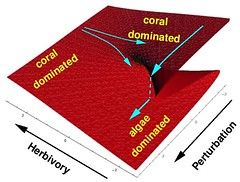A new project involves working with the CEG model and coral reef communities. The main goal is an interactive and instructive module for education, but there’s no reason why the data could not be used for some research also. The exercises are to model the impacts of coral bleaching, and reduction/removal of higher trophic-level fish from the system. Now CEG specifically models potential secondary extinction of species, but it occurs to me that one of the major impacts that we observe on reefs is the decline of corals as dominant or co-dominant benthic cover. This is usually accompanied by an expansion of macroalgae with which the corals compete for space. So the model is being modified to examine the impact of the manipulation of trophic networks (food webs) on the spatial state of the reef (along with secondary extinctions, of course). You can read a bit more about this here.

Assume that the community begins in equlibrium (with regard to spatial competition) at time 0 ($t=0$). If the relative population size of species i is$N_{i}$, then equlibrium is expressed as$K_{i}N_{i}(0) - \sum_{j=1}^{n}N_{j}(0) = 0$
where$K_{i}$ is a competition coefficient (not a constant), and there are n competing species. Therefore,$K_{i}(0) = \frac{\sum_{j=1}^{n}N_{j}(0)}{N_{i}(0)}$
Because population sizes are changing in response to non-competitive factors (trophic), we expect changes to relative population sizes, and hence the coefficient is dynamic. Hence the difference equation governing relative population size during a CEG cascade becomes$N_{i}(t) = \frac{ K_{i}(0) \left [ I_{i}(t) - O_{i}(t) \right ]} {K_{i}(t)}$
where$\frac{K_{i}(0)}{K_{i}(t)} = \frac{\sum_{j=1}^{n}N_{j}(0)}{N_{i}(0)} \frac{N_{i}(t)}{\sum_{j=1}^{n}N_{j}(t)}$$\sum_{j=1}^{n}N_{j}(t)$ is the same for all competitors, and need be computed only once per cascade step.